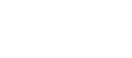# Reynolds number

In fluid mechanics, the Reynolds number (Re) is the ratio of inertial forces to viscous forces and consequently it quantifies the relative importance of these two types of forces for given flow conditions.

It is one of the most important dimensionless numbers in fluid dynamics and is used, usually along with other dimensionless numbers, to provide a criterion for determining dynamic similitude. It is also used to identify and predict different flow regimes, such as laminar or turbulent flow this depends on the pipe diameter, the density and the viscosity of the flowing fluid.

Laminar flow occurs at low Reynolds numbers, where viscous forces are dominant, and is characterised by smooth, constant fluid motion. This occurs when the Reynolds number is less than 2000 and the resistance to flow is independent of the wall roughness.

Turbulent flow occurs when the Reynolds number exceeds 4000. At this time Eddy currents are present within the flow and the ratio of the internal roughness of the pipe to the internal diameter of the pipe needs to be considered to be able to determine the friction factor. In large diameter pipes, the overall effect of the currents is less significant. In small diameter pipes, the internal roughness can have a major influence on the friction factor.

Between the two states (laminar and turbulent) lies the 'critical zone' where the flow is in a process of change, depending upon many possible conditions is unpredictable. It may be considered as a combination of the two flow conditions.Laminar flowColoured filaments are carried along undisturbed by a stream of waterTurbulent flowThe coloured filaments are completely dispersing only a short distance downstream from the point of injection

Reynolds number can be calculated from the following formulaWhen:

Q = flow in L/min
p = weight density of fluid in kg/m3
d = internal pipe diameter in mm
µ = absolute (dynamic) viscosity in centipoise cP